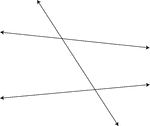### Transversal, 2 Lines Intersected By A

2 lines that are intersected by a third line known as a transversal.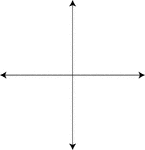### Intersecting Lines, 2 Perpendicular

2 intersecting lines, one of which is vertical and one of which is horizontal. The intersection forms…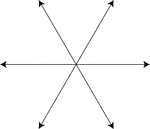### Intersecting Lines, 3

3 lines which intersect at a common point.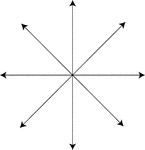### Intersecting Lines, 4

4 lines which intersect at a common point.### Intersecting Lines, 4

4 lines which intersect at a common point.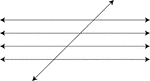### Transversal, 4 Parallel Lines Cut By A

4 parallel lines cut by a 5th line known as the transversal.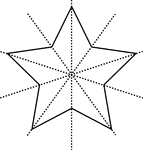### Star, 5-Point

Star with 5 points with lines of symmetry.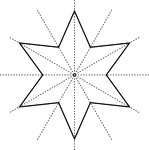### Star, 6-Point

Star with 6 points with lines of symmetry.### Intersecting Lines, 8

8 lines which intersect at a common point.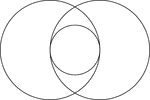### 2 Intersecting Circles

2 congruent circles whose intersection includes a tangent circle with diameter equal to the radii of…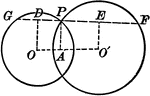### Intersecting Circles

Intersecting circles with chords and radii.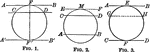### Circles With Parallels Intercepting Equal Arcs

Illustration of a circle with parallels intercepting equal arcs on a circumference.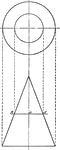### Intersection of a Cone and a Plane

Illustration of the intersection of a cone and a plane.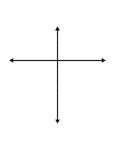### Flashcard of Intersecting Lines

A flashcard featuring an illustration of Intersecting Lines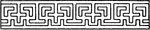### Intersecting Fret Band

The intersecting fret band is repetitive pattern that shows the patterns that cross each other.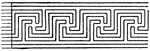### Ordinary Intersecting Fret Band

The ordinary intersecting fret band is a pattern that crosses each other, found on Greek vase paintings.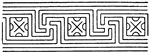### Ordinary Intersecting Fret Band

The ordinary intersecting fret band is a pattern that crosses each other, found on Greek vase paintings.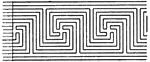### Ordinary Intersecting Fret Band

The ordinary intersecting fret band is a pattern that crosses each other, found on Greek vase paintings.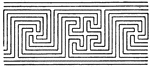### Ordinary Intersecting Fret Band

The ordinary intersecting fret band is a pattern that crosses each other, found on Greek vase paintings.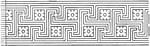### Ordinary Intersecting Fret Band

The ordinary intersecting fret band is a pattern that crosses each other, found on Greek vase paintings.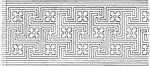### Ordinary Intersecting Fret Band

The ordinary intersecting fret band is a pattern that crosses each other, found on Greek vase paintings.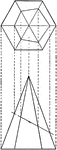### Intersection of Hexagonal Pyramid and a Plane

Illustration of the intersection of a hexagonal pyramid and a plane.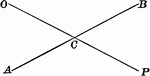### Intersecting Straight Lines

Illustration of two lines intersecting at a point. This can be used to show vertical angles.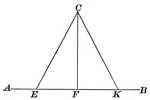### Lines Drawn to Another Line to Form Triangle

Illustration of two straight lines drawn from a point in a perpendicular to a given line, cutting off…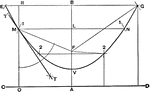### Parabola First Method

Draftsman's first method for drawing a parabola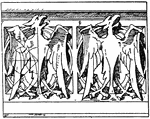### Modern Gothic Parapet

The modern Gothic parapet is a stone design of a wall-like barrier found on the edge of a roof or structure.…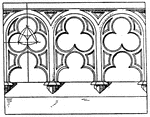### Modern Gothic Parapet

The modern Gothic parapet is a stone design of a wall-like barrier found on the edge of a roof or structure.…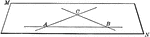### Plane Determined by 2 Intersecting Lines

Illustration of a plane determined by 2 intersecting lines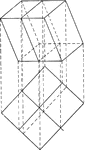### Intersection of a Prism and a Plane

Illustration of the intersection of a prism and a plane.### Intersecting Spheres

Illustration of two intersecting spheres. "The intersection of two spherical surfaces is the circumference…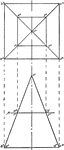### Intersection of Square Pyramid and a Plane

Illustration of the intersection of a square pyramid and a plane.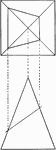### Intersection of Square Pyramid and a Plane

Illustration of the intersection of a square pyramid and a plane.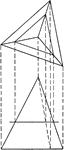### Intersection of Triangular Pyramid and a Plane

Illustration of the intersection of a triangular pyramid and a plane.